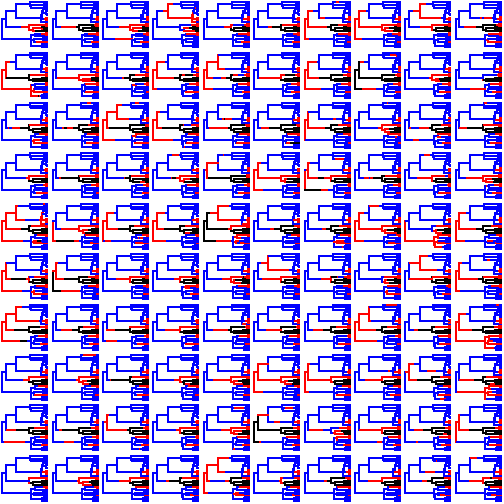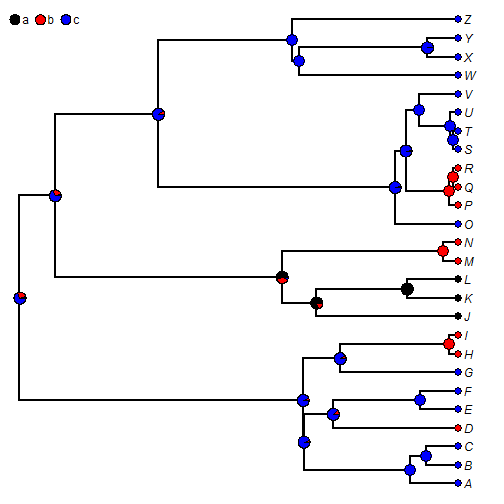## Sunday, April 23, 2017

### Extended S3 `density` method for `"multiSimmap"` object class

I have been trying to rework the S3 `density` method that I recently added to phytools for objects of class `"multiSimmap"`.

This relatively simple method computes (by default) a posterior distribution on the number & types of changes between character states from a set of stochastically mapped histories of a discrete character on the tree.

The implementation in the current CRAN version of phytools, however, permits only binary characters mapped on the tree. In the code below, I have extended this method (& the associated `print` method) for an arbitrary number of character states:

``````## S3 density method for "multiSimmap"
density.multiSimmap<-function(x,...){
if(hasArg(method)) method<-list(...)\$method
else method<-"changes"
if(!method%in%c("densityMap","changes")){
cat("method not recognized. Setting to default method.\n\n")
method<-"changes"
}
if(method=="densityMap") obj<-densityMap(x,plot=FALSE,...)
else if(method=="changes"){
if(hasArg(bw)) bw<-list(...)\$bw
else bw<-1
tmp<-summary(x)
ab<-lapply(2:ncol(tmp\$count),function(i,x) x[,i],x=tmp\$count)
names(ab)<-sapply(strsplit(colnames(tmp\$count)[2:ncol(tmp\$count)],
","),function(x) paste(x,collapse="->"))
ab<-lapply(ab,function(x){
class(x)<-"mcmc"
x })
if(.check.pkg("coda")){
hpd.ab<-lapply(ab,HPDinterval)
} else {
cat("  HPDinterval requires package coda.\n")
cat("  Computing 95% interval from samples only.\n\n")
hpd95<-function(x){
obj<-setNames(c(sort(x)[round(0.025*length(x))],
sort(x)[round(0.975*length(x))]),
c("lower","upper"))
attr(obj,"Probability")<-0.95
obj
}
hpd.ab<-lapply(ab,hpd95)
}
minmax<-range(unlist(ab))
pcalc<-function(x,mm)
hist(x,breaks=seq(mm-1.5,mm+1.5,bw),plot=FALSE)
p.ab<-lapply(ab,pcalc,mm=minmax)
states<-colnames(tmp\$ace)
trans<-names(ab)
obj<-list(hpd=hpd.ab,
p=p.ab,
states=states,trans=trans,
bw=bw,
mins=sapply(ab,min),
meds=sapply(ab,median),
means=sapply(ab,mean),
maxs=sapply(ab,max))
class(obj)<-"changesMap"
}
else if(method=="timings"){
cat("This method doesn't work yet.\n")
obj<-NULL
}
obj
}

print.changesMap<-function(x, ...){
if(hasArg(signif)) signif<-list(...)\$signif
else signif<-2
cat("\nDistribution of changes from stochastic mapping:\n")
NROW<-ceiling(length(x\$trans)/2)
if(NROW>1) cat("\n")
for(i in 1:NROW){
ii<-2*i-1
jj<-ii+1
cat(paste("\t",x\$trans[ii],"\t\t",x\$trans[jj],"\n",sep=""))
cat(paste("\tMin.   :",round(x\$mins[ii],signif),
"\tMin.   :",round(x\$mins[jj],signif),"\n",sep=""))
cat(paste("\tMedian :",round(x\$meds[ii],signif),
"\tMedian :",round(x\$meds[jj],signif),"\n",sep=""))
cat(paste("\tMean   :",round(x\$means[ii],signif),
"\tMean   :",round(x\$means[jj],signif),"\n",sep=""))
cat(paste("\tMax.   :",round(x\$maxs[ii],signif),
"\tMax.   :",round(x\$maxs[jj],signif),"\n\n",sep=""))
}
for(i in 1:length(x\$trans))
cat("95% HPD interval(",x\$trans[i],"): [",x\$hpd[[i]],", ",
x\$hpd[[i]],"]\n",sep="")
cat("\n")
}
``````

Here's an example:

``````library(phytools)
trees
``````
``````## 100 phylogenetic trees with mapped discrete characters
``````
``````par(mfrow=c(10,10))
cols<-setNames(c("black","red","blue"),letters[1:3])
plot(trees,ftype="off",colors=cols)
````````````obj<-density(trees)
obj
``````
``````##
## Distribution of changes from stochastic mapping:
##
##  a->b        a->c
##  Min.   :0   Min.   :0
##  Median :1   Median :0
##  Mean   :0.88    Mean   :0
##  Max.   :3   Max.   :0
##
##  b->a        b->c
##  Min.   :0   Min.   :0
##  Median :1   Median :1
##  Mean   :1.44    Mean   :1.81
##  Max.   :3   Max.   :7
##
##  c->a        c->b
##  Min.   :0   Min.   :2
##  Median :0   Median :4
##  Mean   :0   Mean   :4.48
##  Max.   :0   Max.   :7
##
## 95% HPD interval(a->b): [0, 2]
## 95% HPD interval(a->c): [0, 0]
## 95% HPD interval(b->a): [1, 3]
## 95% HPD interval(b->c): [0, 5]
## 95% HPD interval(c->a): [0, 0]
## 95% HPD interval(c->b): [3, 6]
``````

Note that this breaks the `plot` method associated with this object class, so I will have to get around to fixing that.

Don't forget that phytools also has a `summary` method for this object class, & the results of this summary can be plotted also:

``````obj<-summary(trees)
obj
``````
``````## 100 trees with a mapped discrete character with states:
##  a, b, c
##
## trees have 8.61 changes between states on average
##
## changes are of the following types:
##       a,b a,c  b,a  b,c c,a  c,b
## x->y 0.88   0 1.44 1.81   0 4.48
##
## mean total time spent in each state is:
##              a         b        c    total
## raw  1.0077479 1.3883264 4.882181 7.278256
## prop 0.1384601 0.1907499 0.670790 1.000000
``````
``````plot(obj,colors=cols,ftype="i")